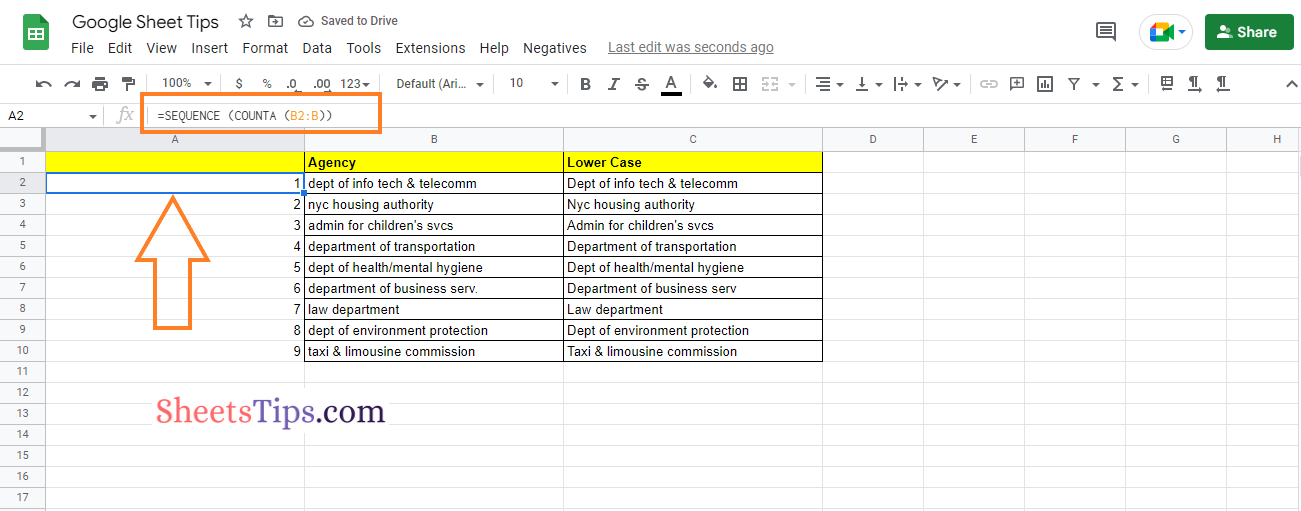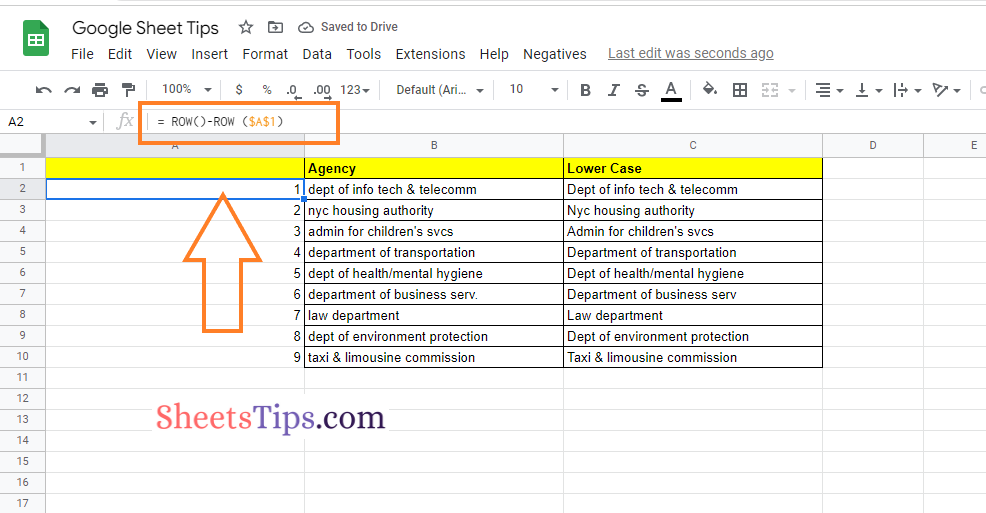# How to Generate Serial Numbers Automatically in Google Sheets – Autonumber in Google Sheets

Google Sheets Sequential Numbers: If you are managing a dataset with records, then it is extremely important for you to have columns with serial numbers. However, entering the serial numbers manually will be a tedious task if you have a large dataset. And to overcome this issue, there are multiple ways in Google Sheets that help us add the serial numbers to the spreadsheet. Let us discuss the ways in which we can auto number in the spreadsheet using Google Sheets Tips. Scroll down to find out more.

## Using the Fill Handle to Row Numbers in Google Sheets

The Fill handle is one of the easiest features that helps to auto generate the serial numbers in the Google Spreadsheet. The detailed steps on how to use the Fill handle for adding a series of numbers to the spreadsheet are explained below:

• 2nd Step: Now on the homepage, move to the cell where you want to add the serial number.
• 3rd Step: Put one in Cell 1 and two in Cell 2. (Make sure you are adding up the numerical values.)
• 4th Step: Select cell 1 and cell 2, and drag the fill handle.

That’s it. Now you will see the fill handle generating the numbered list in the Google Spreadsheet.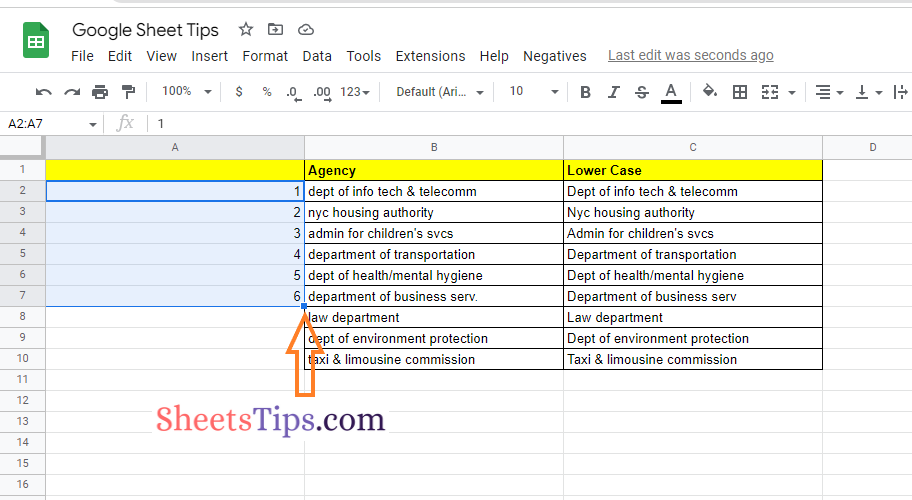### Using a Formula in Google Sheets to Add a Serial Number

The main disadvantage of using the Fill handle feature is that it doesn’t reorder on its own if you delete any of the cells in between. For example, if you have created a serial number with the sequence of 1, 2, and 3, and if you delete the number 2, the sequence would be 1, 3. To overcome this problem, we can simply use the formula method to create a number series in Google Sheets. The detailed steps on how to get this done in the Google Sheets are given below:

• 2nd Step: Now on the homepage, move to the cell where you want to create the serial numbers.
• 3rd Step: Enter the number 1 in cell A1.
• 4th Step: Now in the next cell, enter the formula “=A2+1“. Here, A2 is the cell number. Depending upon your cell number, you may have an alternate cell range here.
• 5th Step: Press the “Return” key and you will find the results as shown in the image given below.

Alternatively, we can also use the formula =SUM (A2,1). Using this formula instead of A2+1 will help to generate the serial numbers.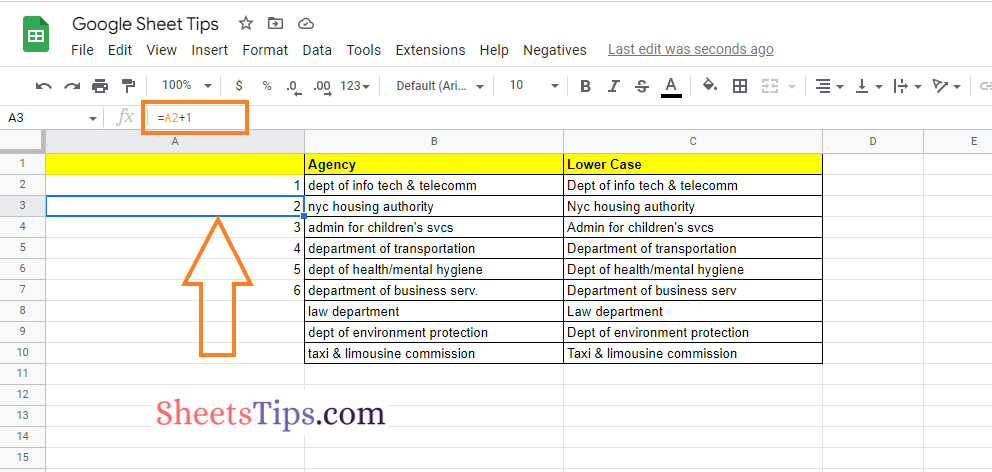### Adding a Serial Number in Google Sheets Using the COUNTA Function

Another easy method to add a serial number to the spreadsheet is to use the COUNTA function. The steps to add a number series using the COUNTA function in Google Sheets are given below.

• 2nd Step: Navigate to the cell where you want to add the serial number.
• 3rd Step: Enter the formula “= COUNTA (\$B\$2:B2)“. Here, B denotes the cell number. Based on your requirements, you can alter the cell number to add the serial number.
• 4th Step: Press the Enter key and you will find the results as shown in the image given below.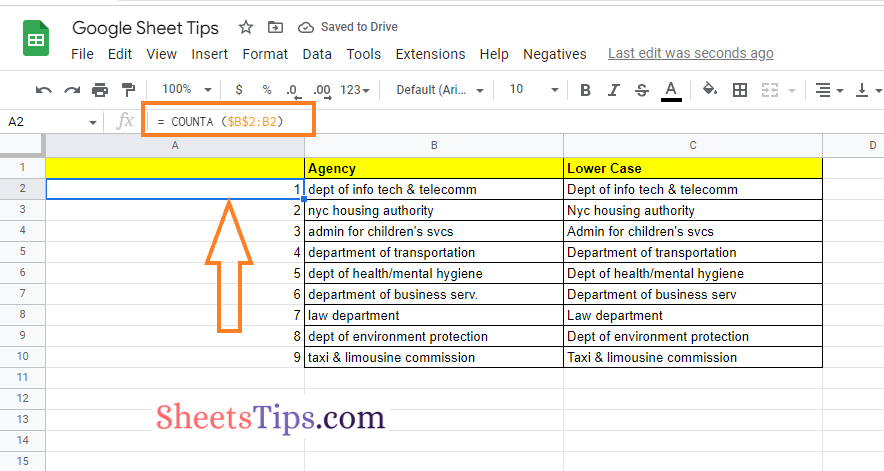### Using the Sequence Function To Add a Serial Number in Google Sheets

Another easy method to add the serial number to the spreadsheet is by using the SEQUENCE function. The steps to add the autocomplete number in Google Sheets using the SEQUENCE function are given below:

• 2nd Step: Now on the homepage, move to the cell where you want to add the number series.
• 3rd Step: Copy and paste =SEQUENCE (COUNTA (B2:B)).
• 4th Step: Press the “Return” key and you will find the results as shown in the image given below.